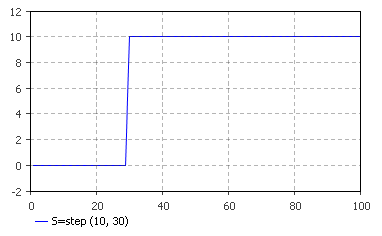AnyLogic

# step

step(double height, double stepTime)

Returns 0 until the stepTime and then returns height.

The plot below illustrates how the function works:#### Parameters

Name Type Description
height double The height of the step.
stepTime double The time of the step.

#### Result

Type Description
double 0 until the stepTime and then returns height.

height — units

stepTime — time

step() — units

## Example

You can study the Multiplier Simul Eqns example model to understand the function usage. The step() function is used in the formula of the GovernmentExpenditure dynamic variable: GovernmentExpenditure = 90 + step( 10, 1 ). If you run the model and switch to the Graphs page, you will see how the step logic affects the system behavior at the time 1.